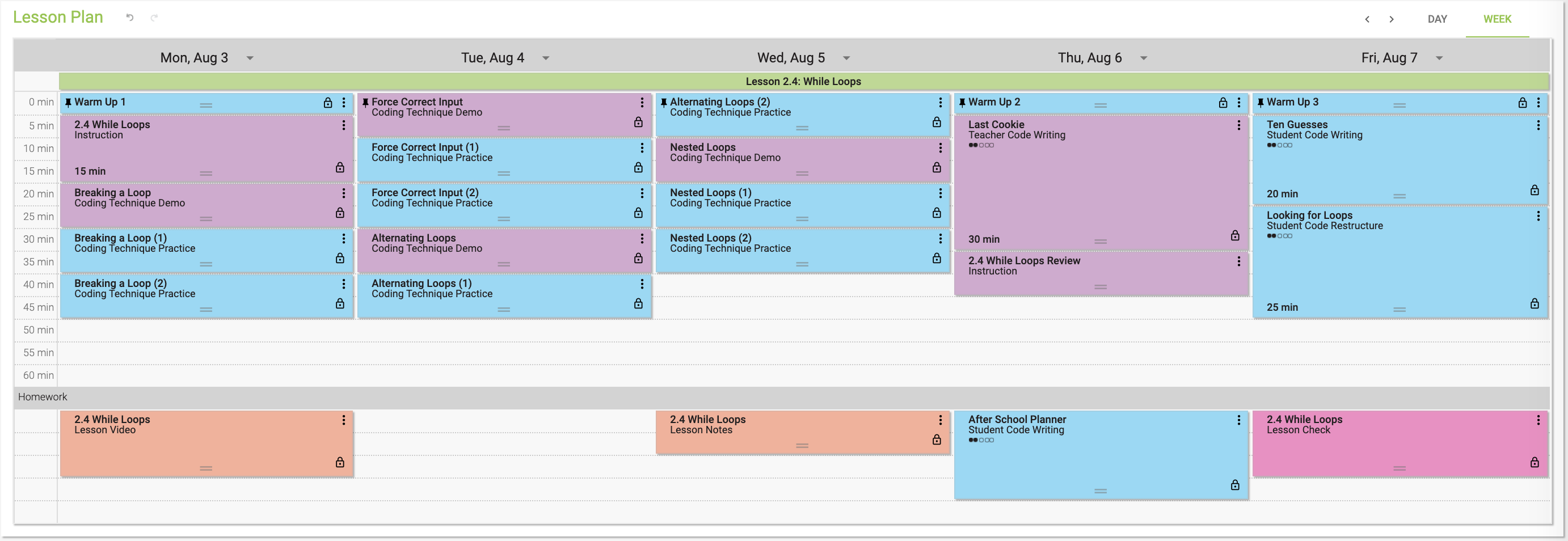CS201 Typical Class Lesson Flow

Let's look at each of the lesson activities in our lesson flow.Warm-up

Each class begins with a warm-up. The warm-up is an “entry-ticket” opening activity that students complete at the start of class. It consists of a short 3-5 line coding exercise that is based on the content from the previous class.

Instruction

Each lesson begins with an instruction lesson activity. This teacher-led whole group activity at the start of every lesson introduces students to the main computer science concepts of the lesson. Students learn key coding vocabularies as you lead them through a small hands-on coding examples.

The lesson then transitions to Coding Techniques

Coding Techniques

For each lesson, we have identified the 3-4 most important coding techniques that students need to learn and  master to achieve fluency related to that lesson topic.

Coding Technique is a specific application of a computer science concept in code. They are usually 5 - 7 lines of codes.

From there, the lesson then moves to students coding larger complete programs, through a Lesson Activity called Coding Exercises

Coding Exercises

These are 20-30 line programs that students code which include all of the CS concepts and coding techniques introduced in this lesson.

We start with a Teacher Coding Writing Exercise (purple), which is a Teacher Whole Group, where you walk the students through coding a complete program, teaching and explaining along the way. Then, students work independently on a Student Code Writing Exercise (blue) , where they code a Coding Exercise on their own, either independently, in a pair with a partner or in a small group.

Lesson Check

The Lesson Check is a 5-7 question formative assessment that is designed to measure students progress toward achieving the Lesson’s learning targets.

We also provide two formative assessments for each unit - Unit Quiz, that is given half way through the unit and an end-of-unit Test.

Code-Your-Own

The Code-Your-Own is an end of lesson capstone project where students code a program of their own design using the Coding Techniques introduced in the lesson. This gives students the opportunity to express their creativity and demonstrate their mastery over the lesson topics and techniques.